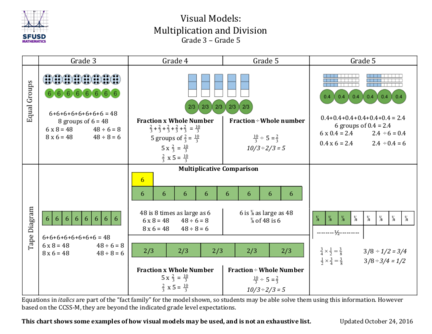# Visual Models of Multiplication and DivisionContributed by:This pdf shows some examples of how visual models may be used to multiply and divide two numbers. It explains the multiplication and division of a fraction with a whole number and the multiplication of 2-digit numbers.
1. Visual Models:
Multiplication and Division
6+6+6+6+6+6+6+6 = 48
Fraction x Whole Number ​Fraction ÷ Whole number 0.4+0.4+0.4+0.4+0.4+0.4 = 2.4
8 groups of 6 = 48
2 2 2 2 2 10 6 groups of 0.4 = 2.4
6 x 8 = 48 48 ÷ 6 = 8 3 +3+3+ 3+3 = 3
6 x 0.4 = 2.4 2.4 ÷ 6 = 0.4
8 x 6 = 48 48 ÷ 8 = 6 5 groups of 23 = 103 10
÷ 5 = 23
3
0.4 x 6 = 2.4 2.4 ÷ 0.4 = 6
5 x 23 = 103 10/3 ÷ 2/3 = 5
2 10
3 x5= 3
Multiplicative Comparison
6
6 6 6 6 6 6 6 6
48 is 8 times as large as 6 6 is ⅛ as large as 48
6 6 6 6 6 6 6 6 6 x 8 = 48 48 ÷ 6 = 8 ⅛ of 48 is 6 ⅛ ⅛ ⅛ ⅛ ⅛ ⅛ ⅛ ⅛
8 x 6 = 48 48 ÷ 8 = 6
--------½---------
6+6+6+6+6+6+6+6 = 48
6 x 8 = 48 48 ÷ 6 = 8 3 1
8 x 6 = 48 48 ÷ 8 = 6 2/3 2/3 2/3 2/3 2/3 4×2 = 38 3/8 ÷ 1/2 = 3/4
1 3
2×4 = 38 3/8 ÷ 3/4 = 1/2

Fraction x Whole Number Fraction ÷ Whole Number
2 10
5x 3 = 3 10 2
3 ÷ 5=3
2 10
3 x5= 3 10/3 ÷ 2/3 = 5
Equations in ​italics are part of the “fact family” for the model shown, so students may be able solve them using this information. However
based on the CCSS-M, they are beyond the indicated grade level expectations.
This chart shows some examples of how visual models may be used, and is not an exhaustive list. ​Updated October 24, 2016
2. Visual Models:
Multiplication and Division
0.4+0.4+0.4+0.4+0.4+0.4 = 2.4
6 groups of 0.4 = 2.4
6 x 0.4 = 2.4 2.4 ÷ 6 = 0.4
6+6+6+6+6+6+6+6 = 48
48-6-6-6-6-6-6-6-6 = 0 0.4 x 6 = 2.4 2.4 ÷ 0.4 = 6
8 groups of 6 = 48
6 x 8 = 48 48 ÷ 6 = 8 ​ Fraction x Whole Number
2 2 2 2 2 10
Fraction ÷ Whole Number
8 x 6 = 48 48 ÷ 8 = 6 3 +3+3+ 3+3 = 3
5 groups of 23 = 103 10
÷ 5 = 23
3
5 x 23 = 103 10/3 ÷ 2/3 = 5
3
2
3 x5= 3
10
4 × 12 = 38 3/8 ÷ 1/2 = 3/4
1
2 × 34 = 38 3/8 ÷ 3/4 = 1/2
Equations in ​italics are part of the “fact family” for the model shown, so students may be able solve them using this information. However
based on the CCSS-M, they are beyond the indicated grade level expectations.
This chart shows some examples of how visual models may be used, and is not an exhaustive list. ​Updated October 24, 2016
3. Visual Models:
Multiplication and Division
0.3 x 0.4 = 0.12 0.12 ÷ 0.3 = 0.4
6 x 8 = 48 48 ÷ 6 = 8
8 x 6 = 48 48 ÷ 8 = 6 0.4 x 0.3 = 0.12 0.12 ÷ 0.4 = 0.3
2-digit x 2-digit ÷ 2-digit Divisor
Whole Numbers Whole Numbers
11 x 13 = 143 143 ÷ 13 = 11
13 x 11 = 143 143 ÷ 11 = 13
2
4 x 13 = 52 52 ÷ 4 = 13 3 x 12 = 2
6 ​2/6 ÷ 1/2 = 2/3
13 x 4 = 52 ​ 52 ÷ 13 = 4 1
2 x 23 = 2
6 ​2/6 ÷ 2/3 = 1/2
0.7 x 1.3 = 0.91 0.91 ÷ 0.7 = 1.3
7 x 138 = 966 966 ÷ 7 = 138 1.3 x 0.7 = 0.91 0.91 ÷ 1.3 = 0.7
138 x 7 = 966 ​966 ÷ 138 = 7
4 x 13 = 52 52 ÷ 4 = 13
13 x 4 = 52 ​ 52 ÷ 13 = 4
14 x 36 = 504 504 ÷ 14 = 36 2 12 x 1 13 = 2 86 = 3 26
36 x 14 = 504 504 ÷ 36 = 14 1 13 x 2 12 = 2 86 = 3 26
Equations in ​italics are part of the “fact family” for the model shown, so students may be able solve them using this information. However
based on the CCSS-M, they are beyond the indicated grade level expectations.
This chart shows some examples of how visual models may be used, and is not an exhaustive list. ​Updated October 24, 2016
4. Visual Models:
Multiplication and Division
Connection to Algorithms: Multiplication (3 x 1 digit)
Fuson, Karen C. and Beckmann, Sybilla. ​Standard Algorithms in the Common Core State Standards. NCSM Journal. Fall/Winter 2012-2013.
This chart shows some examples of how visual models may be used, and is not an exhaustive list. ​Updated October 24, 2016
5. Visual Models:
Multiplication and Division